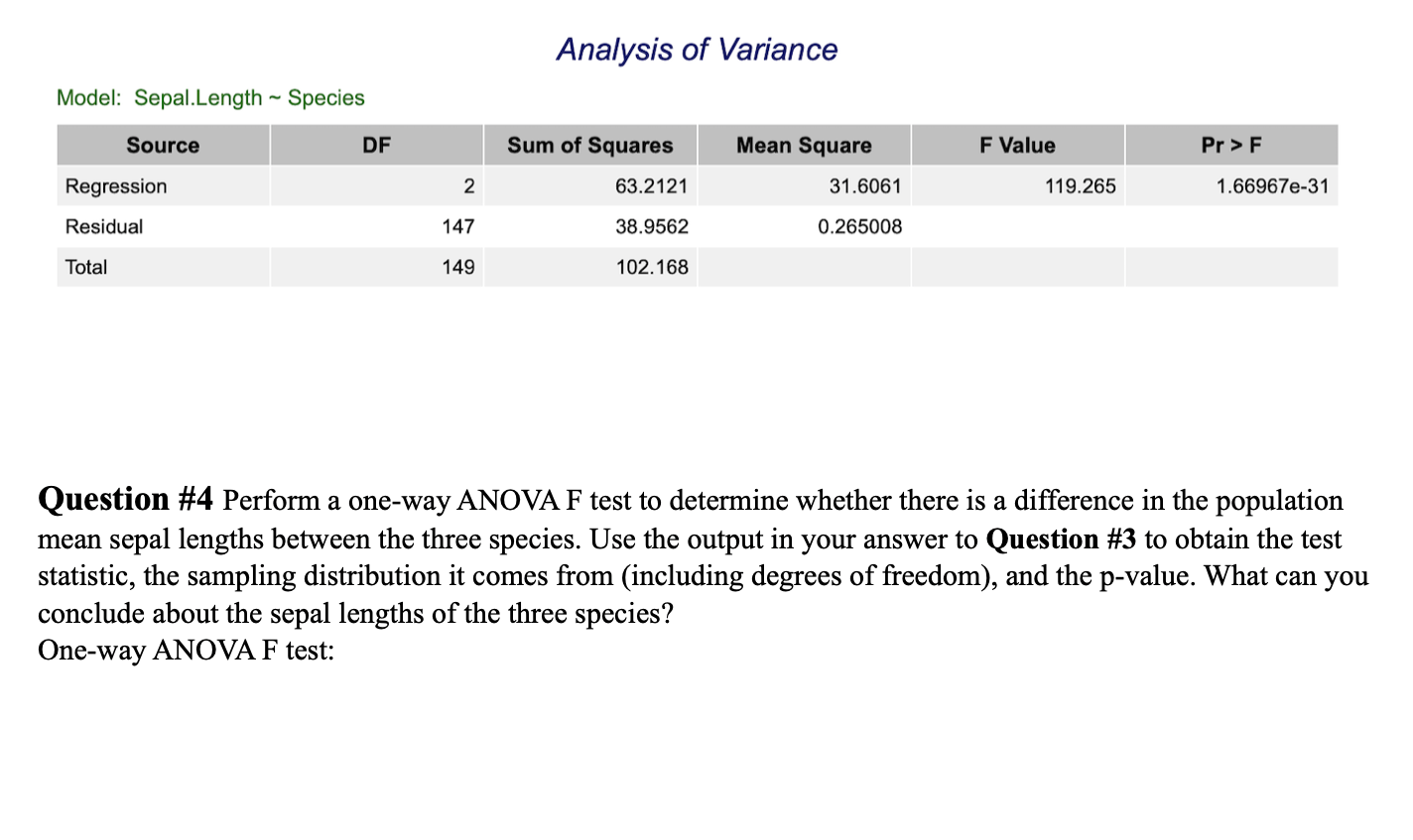Analysis of VarianceModel: Sepal. Length SpeciesF ValueSourceDFSum of SquaresMean SquarePr> F63.212138.9562102.168119.265RegressionResidualTotal214714931.60611.66967e-310.265008Question #4 Perform a one-way ANOVA F test to determine whether there is a difference in the populationmean sepal lengths between the three species. Use the output in your answer to Question #3 to obtain the teststatistic, the sampling distribution it comes from (including degrees of freedom), and the p-value. What can youconclude about the sepal lengths of the three species?One-way ANOVA F test:

Questionhelp_outlineImage TranscriptioncloseAnalysis of Variance Model: Sepal. Length Species F Value Source DF Sum of Squares Mean Square Pr> F 63.2121 38.9562 102.168 119.265 Regression Residual Total 2 147 149 31.6061 1.66967e-31 0.265008 Question #4 Perform a one-way ANOVA F test to determine whether there is a difference in the population mean sepal lengths between the three species. Use the output in your answer to Question #3 to obtain the test statistic, the sampling distribution it comes from (including degrees of freedom), and the p-value. What can you conclude about the sepal lengths of the three species? One-way ANOVA F test: fullscreen
Step 1

One-way ANOVA F test:

The aim is to check whether there is a difference in the population mean sepal length between three species.

The hypotheses to be tested are:

Null hypothesis:

H0: The population mean sepal length for the three species are same.

Alternative hypothesis:

H1: The population mean sepal length for the three species are not same.

Step 2

Observations from the ANOVA table:

The value of the test statistics is F = 119.265.

The p-value for the test is 1.66967×10-31.

The sampling distribution is F distribution with degrees of freedom (2,147)

Step 3

Decision Rule:

Assume the level of significance is 0.05. If the p-value is less than 0.05, then reject the null hypothesis. Otherwise fail to reject the null hypothesis.

Conclusion:

From the output, the p-value is 1.66967×10-31. This p-value is less than the level of significance, that is, p-value (=1.66967...

Want to see the full answer?

See Solution

Want to see this answer and more?

Our solutions are written by experts, many with advanced degrees, and available 24/7

See Solution
Tagged in

Other